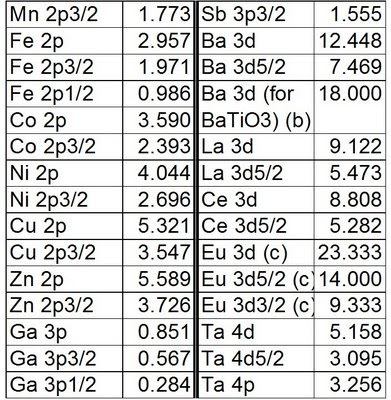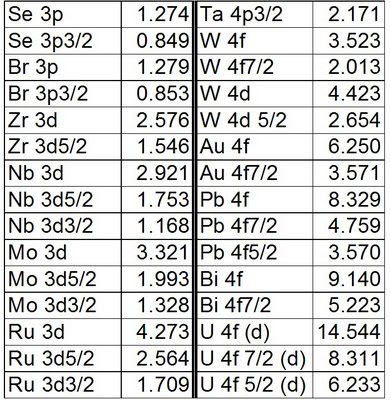### Relative Sensitivity Factors (R.S.F.)

In some instances, different portions of the various core levels (other than those normally used) need to be used for quantification. For example: when overlaps of lines from different species are present it may be necessary to quantify using only the 2p3/2 line instead of the total 2p lineshape. Table 1 presents common relative sensitivity factor (R.S.F.) values derived for CasaXPS based on R.S.F. values used for the Kratos AXIS Supra, Ultra and Nova (this may differ from your instrument - check your library values versus the standard values listed (example: 2p, 3d, 4f, F 1s = 1) before using the derived values (example: 2p3/2, 3d5/2, 4f7/2)). Values that have been derived for specific cases based on standard samples are also presented (example: Mg KLL, Ba for BaTiO3, Eu 3d and U 4f).

To calculate a new R.S.F. for other elements/core lines (for the R.S.F. table type used by the CasaXPS/ Kratos Supra/Ultra/Nova combination) the following formula are used.

RSFp3/2 = RSFp * 2/3

RSFp1/2 = RSFp * 1/3

RSFd5/2 = RSFd * 3/5

RSFd3/2 = RSFd * 2/5

RSFf7/2 = RSFf * 4/7

RSFf5/2 = RSFf * 3/7Table 1. Relative sensitivity factors for CasaXPS / Kratos Supra  / Ultra / Nova.

a) Use a window of 298-300eV (see Mg link for more details)

b) Calculated from BaTiO3 standard sample

c) New value from standard sample

d) Calculated values based on RSF's from SSX-100 XPS (U 4f = 48.86, Th 4f= 42.75) ratiod to Th 4f from Kratos instruments (Th 4f = 12.75). 48.86/42.75 = RSF(U 4f Kratos)/12.75; RSF(U 4f Kratos) = 14.544. It would be useful if this value could be confirmed with a well characterized UO2 sample.